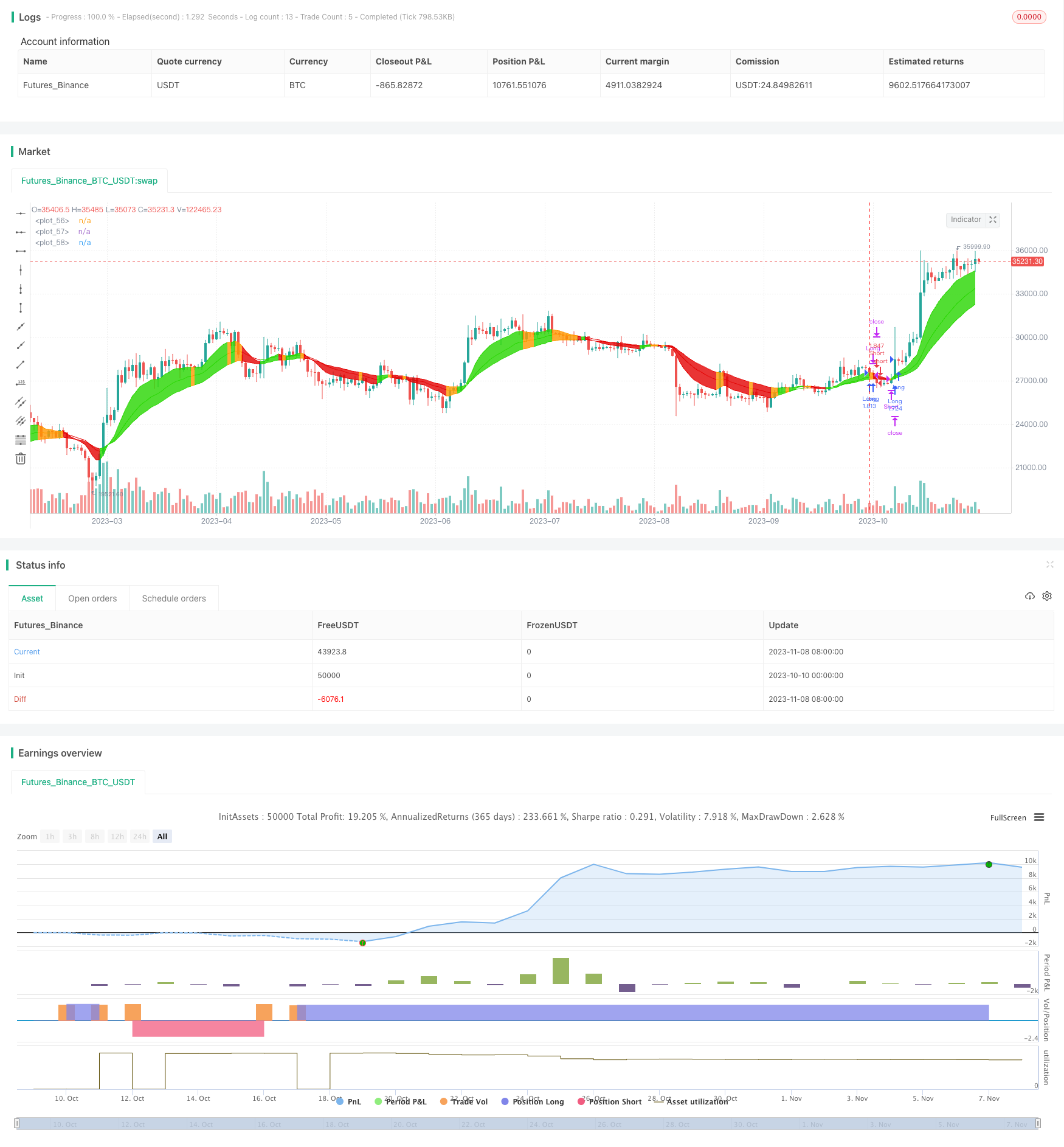# Three EMA Trend Following Strategy

Author: ChaoZhang, Date: 2023-11-10 11:45:30
Tags:## Overview

The Three EMA trend following strategy judges the price trend direction by calculating EMA lines of different periods, and follows the trend automatically. This strategy is simple and effective, especially in trending instruments.

## Strategy Logic

This strategy calculates three EMA lines with different periods, specifically 10-period, 20-period and 30-period EMA. The ema function in code generates the three EMA lines.

The core logic is to judge the direction consistency of the three EMA lines. If all three EMA lines rise together, a long signal is generated. If all three lines fall together, a short signal is generated.

Specifically, if ema1, ema2 and ema3 all rise in the last bar, enter_long becomes true and a long signal is generated. If ema1, ema2 and ema3 all fall in the last bar, enter_short becomes true and a short signal is generated.

Based on the long and short signals, the strategy will open corresponding long and short positions. The exit logic is opposite to entry signals. If ema1, ema2 and ema3 don’t rise together in current bar, exit_long becomes true and long position will be closed. If ema1, ema2 and ema3 don’t fall together in current bar, exit_short becomes true and short position will be closed.

By judging the direction consistency of the three EMA lines, the overall trend can be determined and followed.

• Using three EMA lines can judge the trend direction more reliably compared to a single line. The probability of wrong signals is lower.

• EMA is more sensitive to price changes and can reflect trend reversal in time. It is more suitable for trend judgment compared to SMA etc.

• The combination of different period EMA takes both short-term and mid-long term trend into consideration. 10-period EMA for short-term, 20 and 30-period EMA for mid-long term trend.

• The strategy logic is simple and easy to understand, suitable for beginners. Also the parameters have large optimization space for different instruments.

• The strategy is solely based on EMA lines, requiring less resources and suitable for high concurrency.

## Risks

• EMA line direction consistency is necessary but insufficient for trend judgment. Wrong signals may occur during EMA line false breakout.

• EMA lines lag in trend reversal, unable to reflect turning points in time, which may cause losses.

• EMA is sensitive to price changes, frequent long-short position flip may increase transaction costs.

• The strategy is ineffective in ranging, volatile market where EMA lines fluctuate frequently.

• Can optimize EMA period difference to reduce false signals. Or add other indicators to filter fake breakouts.

• Add momentum indicators to confirm real trend and identify turning points, reducing losses. Also can loosen stop loss.

• Increase EMA periods to reduce position flip frequency. Or use other MA indicators.

• Suspend strategy when ranging market is identified, avoiding unnecessary trades.

## Optimization

• Add filters: Add MA, BOLL etc to avoid EMA fake breakouts.

• Stop loss: Trailing stop to lock profits.

• Risk management: Optimize position sizing to limit single loss impact.

• Market regime: Use volatility to gauge oscillation and control strategy engagement.

• Adaptive parameters: Auto-optimize EMA periods based on market changes to improve robustness.

## Conclusion

The Three EMA trend following strategy trades by identifying trend direction via EMA lines. It is simple and practical with large optimization space. Risks like false breakouts and oscillation should be noted. With continuous optimizations, this strategy can become a robust trend following solution.

```/*backtest
start: 2023-10-10 00:00:00
end: 2023-11-09 00:00:00
period: 1d
basePeriod: 1h
exchanges: [{"eid":"Futures_Binance","currency":"BTC_USDT"}]
*/

// This source code is subject to the terms of the Mozilla Public License 2.0 at https://mozilla.org/MPL/2.0/

//@version=4
strategy("PMA Strategy Idea",
shorttitle="PMA",
overlay=true,
pyramiding=0,
default_qty_type=strategy.percent_of_equity,
default_qty_value=100,
initial_capital=1000,
commission_type=strategy.commission.percent,
commission_value=0.075)

// ____ Inputs

ema1_period = input(title="EMA1 Period", defval=10)
ema2_period = input(title="EMA2 Period", defval=20)
ema3_period = input(title="EMA3 Period", defval=30)
long_only = input(title="Long Only", defval=false)
slp = input(title="Stop-loss (%)", minval=1.0, maxval=25.0, defval=5.0)
use_sl = input(title="Use Stop-Loss", defval=false)

// ____ Logic

ema1 = ema(hlc3, ema1_period)
ema2 = ema(hlc3, ema2_period)
ema3 = ema(hlc3, ema3_period)

enter_long = (rising(ema1, 1) and rising(ema2, 1) and rising(ema3, 1))
exit_long = not enter_long
enter_short = (falling(ema1, 1) and falling(ema2, 1) and falling(ema3, 1))
exit_short = not enter_short

strategy.entry("Long", strategy.long, when=enter_long)
strategy.close("Long", when=exit_long)
if (not long_only)
strategy.entry("Short", strategy.short, when=enter_short)
strategy.close("Short", when=exit_short)

// ____ SL

sl_long = strategy.position_avg_price * (1- (slp/100))
sl_short = strategy.position_avg_price * (1 + (slp/100))
if (use_sl)
strategy.exit(id="SL", from_entry="Long", stop=sl_long)
strategy.exit(id="SL", from_entry="Short", stop=sl_short)

// ____ Plots

colors =
enter_long ? #27D600 :
enter_short ? #E30202 :
color.orange

ema1_plot = plot(ema1, color=colors)
ema2_plot = plot(ema2, color=colors)
ema3_plot = plot(ema3, color=colors)
fill(ema1_plot, ema3_plot, color=colors, transp=50)

```

More